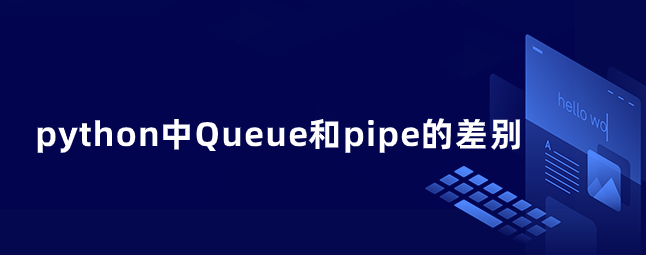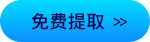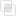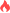python中Queue和pipe的差别2021-03-05 17:06:051058浏览 · 0收藏 · 0评论1、区别

（1）Queue使用 putget维护队列 ，pipe使用 send recv维护队列 。

（2）pipe只提供两个端点，而Queue没有限制。

（3）Queue的封装比较好，Queue只提供一个结果，可以被多个进程同时调用；Pipe()返回两个结果，分别由两个进程调用。

（4）Queue的实现基于pipe，所以pipe的运行速度比Queue快很多

（5）当只需要两个进程时，管道更快，当需要多个进程同时操作队列时，使用队列。

2、实例

```import random
import time
from multiprocessing import Process, Pipe, current_process
def produce(conn):
while True:
new = random.randint(0, 100)
print('{} produce {}'.format(current_process().name, new))
conn.send(new)
time.sleep(random.random())
def consume(conn):
while True:
print('{} consume {}'.format(current_process().name, conn.recv()))        time.sleep(random.random())
if __name__ == '__main__':
pipe = Pipe()
p1 = Process(target=produce, args=(pipe,))
p2 = Process(target=consume, args=(pipe,))
p1.start()
p2.start()```python中sys模块怎么用？python中协程的关闭与异常python实现异步的两种框架python异步中selectors的使用python多线程中join的应用场景python进程池的使用注意python多进程与多线程的简单区分python进程的交流方式python分数如何使用879

python tempfile创建文件382

python finally语句如何使用206